Open In App
Related Articles
• RD Sharma Class 9 Solutions

# Class 9 RD Sharma Solutions – Chapter 8 Introduction to Lines and Angles- Exercise 8.4 | Set 1

### Question 1: In figure, AB, CD and ∠1 and ∠2 are in the ratio 3 : 2. Determine all angles from 1 to 8.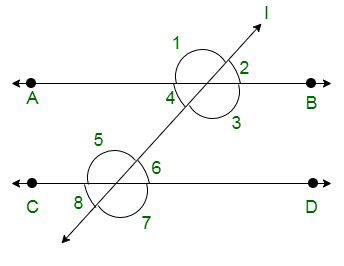Solution:

Assume,

∠1 = 3x and ∠2 = 2x

From the following figure: ∠1 and ∠2 are a linear pair of angles

Thus,

∠1 + ∠2 = 180°

3x + 2x = 180°

5x = 180°

x =x = 36°

Hence,

∠1 = 3x = 108°

and

∠2 = 2x = 72°

We know that:

(Vertically opposite angles are equal)

Here the pairs of vertically opposite angles are:

(∠1 = ∠3);

(∠2 = ∠4);

(∠5, ∠7)

and

(∠6 = ∠8)

∠1 = ∠3 = 108°

∠2 = ∠4 = 72°

∠5 = ∠7

∠6 = ∠8

Now as it is known

(if a transversal intersects any parallel lines, then the corresponding angles are equal)

∠1 = ∠5 = ∠7 = 108°

∠2 = ∠6 = ∠8 = 72°

Hence,

∠1 = 108°, ∠2 = 72°, ∠3 = 108°, ∠4 = 72°, ∠5 = 108°, ∠6 = 72°, ∠7 = 108° and ∠8 = 72°

### Question 2: In figure, I, m, and n are parallel lines intersected by transversal p at X, Y and Z respectively. Find ∠1, ∠2, and ∠3.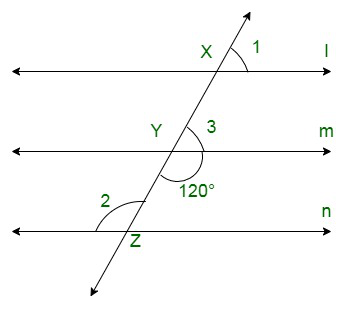Solution:

Here as given in the figure

∠Y = 120° {Vertical opposite angles]}

∠3 + ∠Y = 180° {Linear pair angles}

∠3= 180 – 120

⇒ ∠3= 60°

As we can see the line ‘l’ is parallel to line ‘m’,

∠1 = ∠3 {Corresponding angles}

∠1 = 60°

Now, line ‘m’ is parallel to line ‘n’,

∠2 = ∠Y {Alternate interior angles}

∠2 = 120°

∠1 = 60°,

∠2 = 120°

and

∠3 = 60°.

### Question 3: In figure, AB || CD || EF and GH || KL. Find ∠HKL.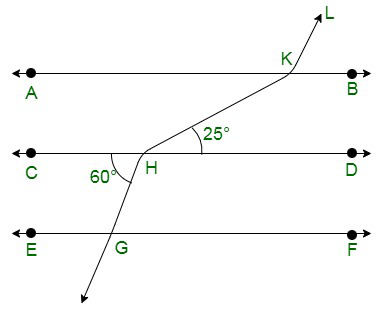Solution:

Construct: Extend LK to meet line GF at point P.

As shown below.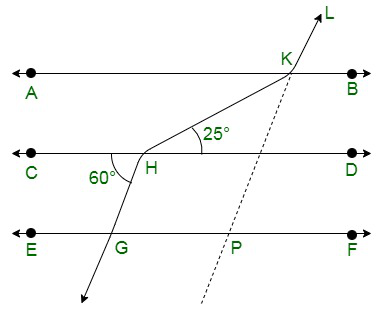Here as from the figure,

CD || GF,

∠CHG =∠HGP = 60° {alternate angles}

∠HGP =∠KPF = 60° {Corresponding angles of parallel lines}

Thus,

∠KPG =180° – 60°

⇒∠KPG = 120°

∠GPK = ∠AKL= 120° {Corresponding angles of parallel lines}

∠AKH = ∠KHD = 25° {alternate angles of parallel lines}

Thus,

∠HKL = ∠AKH + ∠AKL

⇒25 + 120

⇒∠HKL = 145°

### Question 4: In figure, show that AB || EF.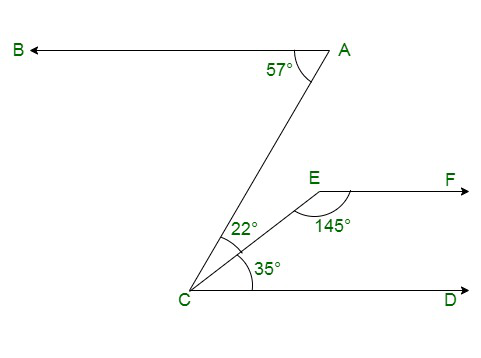Solution:

Construct: Produce EF to intersect AC at point N.

As it is seen in the figure: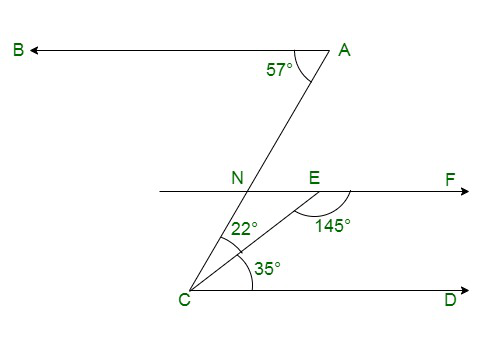∠BAC = 57°

and

∠ACD = 22°+35° = 57°

{Alternative angles of parallel lines are equal}

BA || EF …..(i)

We know that,

Sum of Co-interior angles of parallel lines is 180°

EF || CD

∠DCE + ∠CEF = 35 + 145 = 180° …(ii)

From (i) and (ii)

AB || EF {Lines parallel to the same line are parallel to each other}

Hence Proved.

### Question 5: In figure, if AB || CD and CD || EF, find ∠ACE.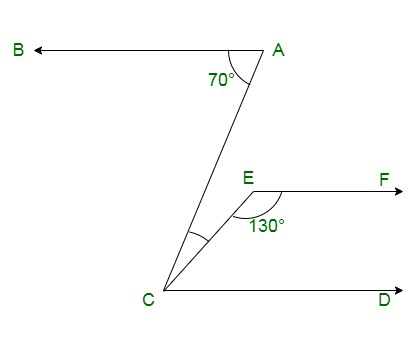Solution:

Given:

CD || EF

∠ FEC + ∠ECD = 180° {Sum of co-interior angles is supplementary to each other}

∠ECD = 180° – 130° = 50°

Now, BA || CD

∠BAC = ∠ACD = 70° {Alternative angles of parallel lines are equal}

Thus,

∠ACE + ∠ECD =70°

∠ACE = 70° – 50°

∠ACE = 20°

### Question 6: In figure, PQ || AB and PR || BC. If ∠QPR = 102°, determine ∠ABC. Give reasons.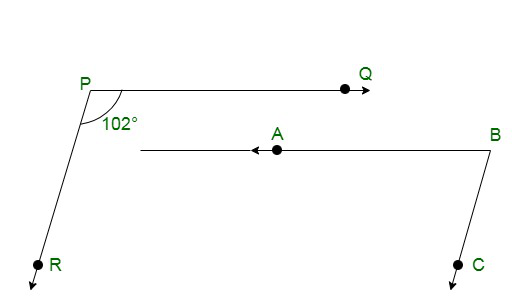Solution:

Construct:

Extend line AB to meet line PR at point G.

As shown below;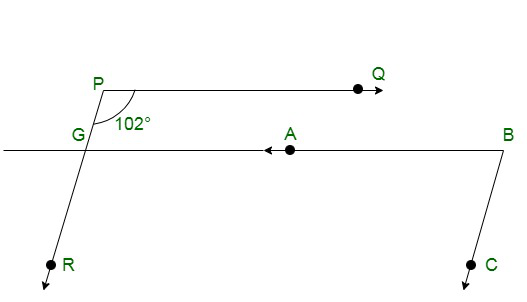Given:

PQ || AB,

∠QPR = ∠BGR =102° {Corresponding angles of parallel lines}

And

PR || BC,

∠RGB+ ∠CBG =180° {Corresponding angles are supplementary}

∠CBG = 180° – 102° = 78°

Thus,

∠CBG = ∠ABC

⇒ ∠ABC = 78°

### Question 7: In figure, state which lines are parallel and why?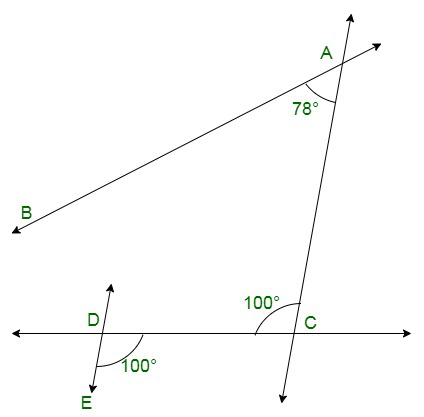Solution:

As we know that,

If a transversal intersects two lines such that a pair of alternate interior angles are equal, then the two lines are parallel

As we can see from the figure:

⇒ ∠EDC = ∠DCA = 100°

Lines DE and AC are intersected by a transversal DC such that the pair of alternate angles are equal.

Hence,

DE || AC

### Question 8: In figure, if l||m, n || p and ∠1 = 85°, find ∠2.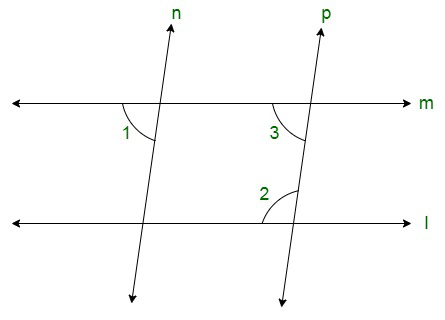Solution:

Given:

∠1 = 85°

We know that,

When a line cuts the parallel lines, the pair of alternate interior angles are equal.

⇒ ∠1 = ∠3 = 85°

Thus again, co-interior angles are supplementary,

Therefore

∠2 + ∠3 = 180°

∠2 + 55° =180°

∠2 = 180° – 85°

∠2 = 95°

### Question 9: If two straight lines are perpendicular to the same line, prove that they are parallel to each other.

Solution:

Assume lines ‘l’ and ‘m’ are perpendicular to ‘n’,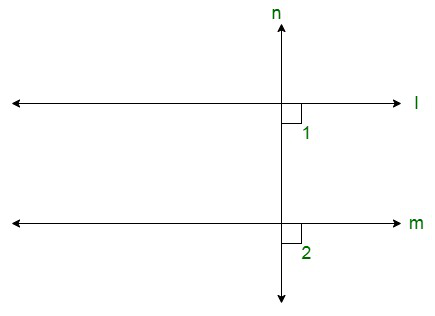Thus

∠1= ∠2=90°

Therefore,

The lines ‘l’ and ‘m’ are cut by a transversal line i.e. ‘n’

The corresponding angles are equal, so it can be seen that,

Line ‘l’ is parallel to line ‘m’.

### Question 10: Prove that if the two arms of an angle are perpendicular to the two arms of another angle, then the angles are either equal or supplementary.

Solution:

Consider the angles be ∠ACB and ∠ABD

Let AC perpendicular to AB, and

CD is perpendicular to BD.

To Prove:

∠ACD = ∠ABD

∠ACD + ∠ABD =180°

Proof :

∠A+ ∠C+ ∠D+ ∠B = 360° {Sum of angles of quadrilateral is 360°}

180° + ∠C + ∠B = 360°

∠C + ∠B = 360° –180°

Thus,

∠ACD + ∠ABD = 180°

And

∠ABD = ∠ACD = 90°

Therefore, angles are equal as well as supplementary.